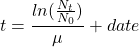# How long do I need to get enough cells?

Usually, we need a defined number of cells for our experiments or for the generation of a cell bank. But how long do we need for this? If we know our cells it is quite easy to calculate the day of harvest. All we need is the number of cells available at the beginning (N0), the number of cells we will need in the end (Nt) and the growth rate µ. (See calculator for growth rates.)

You would like to know how the values are calculated? –  Have a look at the equation.

μ = growth rate [1/d]

N0 = number of viable cells

Nt = number of cells needed

t = day of harvest [date]